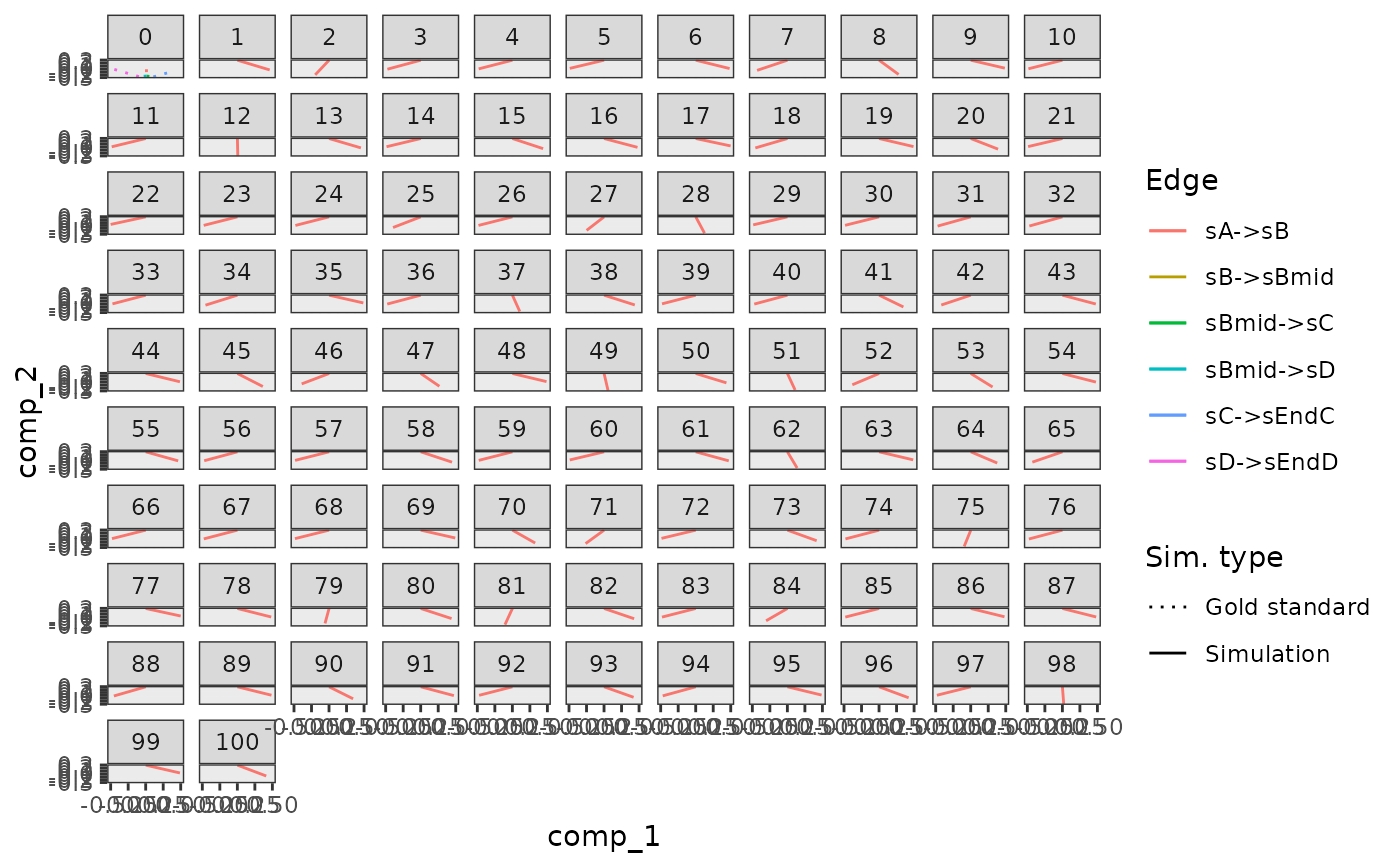Visualise the mapping of the simulations to the gold standard

plot_gold_mappings(
model,
selected_simulations = NULL,
do_facet = TRUE,
mapping = aes_string("comp_1", "comp_2")
)

## Arguments

model A dyngen intermediary model for which the simulations have been run with generate_cells(). Which simulation indices to visualise. Whether or not to facet according to simulation index. Which components to plot.

## Value

A ggplot2 object.

## Examples

# \donttest{
data("example_model")
plot_gold_mappings(example_model)# }